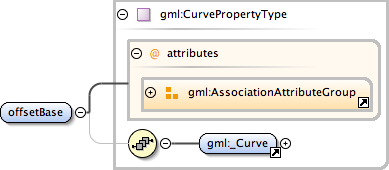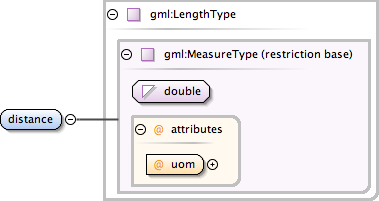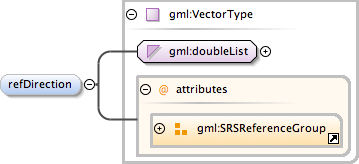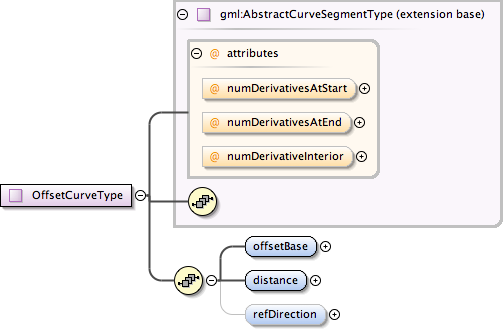### Showing:

 Annotations Attributes Diagrams Instances Properties Source Used by
Element gml:OffsetCurveType / gml:offsetBase
Namespace http://www.opengis.net/gml
Annotations
 ```offsetBase is a reference to thecurve from which this curve is define as an offset.```
DiagramType gml:CurvePropertyType
Properties
 content: complex
Model gml:_Curve
Children gml:_Curve
Instance
 ``` {1,1} ```
Attributes
QName Type Fixed Default Use Annotation
gml:remoteSchema anyURI optional
 `Reference to an XML Schema fragment that specifies the content model of the propertys value. This is in conformance with the XML Schema Section 4.14 Referencing Schemas from Elsewhere.`
 ```The 'actuate' attribute is used to communicate the desired timing of traversal from the starting resource to the ending resource; it's value should be treated as follows: onLoad - traverse to the ending resource immediately on loading the starting resource onRequest - traverse from the starting resource to the ending resource only on a post-loading event triggered for this purpose other - behavior is unconstrained; examine other markup in link for hints none - behavior is unconstrained```
 ```The 'show' attribute is used to communicate the desired presentation of the ending resource on traversal from the starting resource; it's value should be treated as follows: new - load ending resource in a new window, frame, pane, or other presentation context replace - load the resource in the same window, frame, pane, or other presentation context embed - load ending resource in place of the presentation of the starting resource other - behavior is unconstrained; examine other markup in the link for hints none - behavior is unconstrained```
Source
 ``` offsetBase is a reference to thecurve from which this curve is define as an offset. ```
Schema location http://schemas.opengis.net/gml/3.1.1/base/geometryPrimitives.xsd
Element gml:OffsetCurveType / gml:distance
Namespace http://www.opengis.net/gml
Annotations
 ```distance is the distance at which the offset curve is generated from the basis curve. In 2D systems, positive distances are to be to the left of the basis curve, and the negative distances are to be to the right of the basis curve.```
DiagramType gml:LengthType
Type hierarchy
Properties
 content: complex
Attributes
QName Type Fixed Default Use Annotation
uom anyURI required
Source
 ``` distance is the distance at which the offset curve is generated from the basis curve. In 2D systems, positive distances are to be to the left of the basis curve, and the negative distances are to be to the right of the basis curve. ```
Schema location http://schemas.opengis.net/gml/3.1.1/base/geometryPrimitives.xsd
Element gml:OffsetCurveType / gml:refDirection
Namespace http://www.opengis.net/gml
Annotations
 ```refDistance is used to define the vector direction of the offset curve from the basis curve. It can be omitted in the 2D case, where the distance can be positive or negative. In that case, distance defines left side (positive distance) or right side (negative distance) with respect to the tangent to the basis curve. In 3D the basis curve shall have a well defined tangent direction for every point. The offset curve at any point in 3D, the basis curve shall have a well-defined tangent direction for every point. The offset curve at any point (parameter) on the basis curve c is in the direction - - - - s = v x t where v = c.refDirection() and - t = c.tangent() - For the offset direction to be well-defined, v shall not on any point of the curve be in the same, or opposite, direction as - t. The default value of the refDirection shall be the local co-ordinate axis vector for elevation, which indicates up for the curve in a geographic sense. NOTE! If the refDirection is the positive tangent to the local elevation axis ("points upward"), then the offset vector points to the left of the curve when viewed from above.```
DiagramType gml:VectorType
Type hierarchy
Properties
 content: complex minOccurs: 0
Attributes
QName Type Fixed Default Use Annotation
axisLabels gml:NCNameList optional
 ```Ordered list of labels for all the axes of this CRS. The gml:axisAbbrev value should be used for these axis labels, after spaces and forbiddden characters are removed. When the srsName attribute is included, this attribute is optional. When the srsName attribute is omitted, this attribute shall also be omitted.```
srsDimension positiveInteger optional
 ```The "srsDimension" is the length of coordinate sequence (the number of entries in the list). This dimension is specified by the coordinate reference system. When the srsName attribute is omitted, this attribute shall be omitted.```
srsName anyURI optional
 ```In general this reference points to a CRS instance of gml:CoordinateReferenceSystemType (see coordinateReferenceSystems.xsd). For well known references it is not required that the CRS description exists at the location the URI points to. If no srsName attribute is given, the CRS must be specified as part of the larger context this geometry element is part of, e.g. a geometric element like point, curve, etc. It is expected that this attribute will be specified at the direct position level only in rare cases.```
uomLabels gml:NCNameList optional
 ```Ordered list of unit of measure (uom) labels for all the axes of this CRS. The value of the string in the gml:catalogSymbol should be used for this uom labels, after spaces and forbiddden characters are removed. When the axisLabels attribute is included, this attribute shall also be included. When the axisLabels attribute is omitted, this attribute shall also be omitted.```
Source
 ``` refDistance is used to define the vector direction of the offset curve from the basis curve. It can be omitted in the 2D case, where the distance can be positive or negative. In that case, distance defines left side (positive distance) or right side (negative distance) with respect to the tangent to the basis curve. In 3D the basis curve shall have a well defined tangent direction for every point. The offset curve at any point in 3D, the basis curve shall have a well-defined tangent direction for every point. The offset curve at any point (parameter) on the basis curve c is in the direction - - - - s = v x t where v = c.refDirection() and - t = c.tangent() - For the offset direction to be well-defined, v shall not on any point of the curve be in the same, or opposite, direction as - t. The default value of the refDirection shall be the local co-ordinate axis vector for elevation, which indicates up for the curve in a geographic sense. NOTE! If the refDirection is the positive tangent to the local elevation axis ("points upward"), then the offset vector points to the left of the curve when viewed from above. ```
Schema location http://schemas.opengis.net/gml/3.1.1/base/geometryPrimitives.xsd
Complex Type gml:OffsetCurveType
Namespace http://www.opengis.net/gml
Annotations
 ```An offset curve is a curve at a constant distance from the basis curve. They can be useful as a cheap and simple alternative to constructing curves that are offsets by definition.```
DiagramType extension of gml:AbstractCurveSegmentType
Type hierarchy
Used by
 Element gml:OffsetCurve
Model gml:offsetBase , gml:distance , gml:refDirection{0,1}
Children gml:distance, gml:offsetBase, gml:refDirection
Attributes
QName Type Fixed Default Use Annotation
numDerivativeInterior integer 0 optional
 ```The attribute "numDerivativesInterior" specifies the type of continuity that is guaranteed interior to the curve. The default value of "0" means simple continuity, which is a mandatory minimum level of continuity. This level is referred to as "C 0 " in mathematical texts. A value of 1 means that the function and its first derivative are continuous at the appropriate end point: "C 1 " continuity. A value of "n" for any integer means the function and its first n derivatives are continuous: "C n " continuity. NOTE: Use of these values is only appropriate when the basic curve definition is an underdetermined system. For example, line string segments cannot support continuity above C 0 , since there is no spare control parameter to adjust the incoming angle at the end points of the segment. Spline functions on the other hand often have extra degrees of freedom on end segments that allow them to adjust the values of the derivatives to support C 1 or higher continuity.```
numDerivativesAtEnd integer 0 optional
 ```The attribute "numDerivativesAtEnd" specifies the type of continuity between this curve segment and its successor. If this is the last curve segment in the curve, one of these values, as appropriate, is ignored. The default value of "0" means simple continuity, which is a mandatory minimum level of continuity. This level is referred to as "C 0 " in mathematical texts. A value of 1 means that the function and its first derivative are continuous at the appropriate end point: "C 1 " continuity. A value of "n" for any integer means the function and its first n derivatives are continuous: "C n " continuity. NOTE: Use of these values is only appropriate when the basic curve definition is an underdetermined system. For example, line string segments cannot support continuity above C 0 , since there is no spare control parameter to adjust the incoming angle at the end points of the segment. Spline functions on the other hand often have extra degrees of freedom on end segments that allow them to adjust the values of the derivatives to support C 1 or higher continuity.```
numDerivativesAtStart integer 0 optional
 ```The attribute "numDerivativesAtStart" specifies the type of continuity between this curve segment and its predecessor. If this is the first curve segment in the curve, one of these values, as appropriate, is ignored. The default value of "0" means simple continuity, which is a mandatory minimum level of continuity. This level is referred to as "C 0 " in mathematical texts. A value of 1 means that the function and its first derivative are continuous at the appropriate end point: "C 1 " continuity. A value of "n" for any integer means the function and its first n derivatives are continuous: "C n " continuity. NOTE: Use of these values is only appropriate when the basic curve definition is an underdetermined system. For example, line string segments cannot support continuity above C 0 , since there is no spare control parameter to adjust the incoming angle at the end points of the segment. Spline functions on the other hand often have extra degrees of freedom on end segments that allow them to adjust the values of the derivatives to support C 1 or higher continuity.```
Source
 ``` An offset curve is a curve at a constant distance from the basis curve. They can be useful as a cheap and simple alternative to constructing curves that are offsets by definition. offsetBase is a reference to thecurve from which this curve is define as an offset. distance is the distance at which the offset curve is generated from the basis curve. In 2D systems, positive distances are to be to the left of the basis curve, and the negative distances are to be to the right of the basis curve. refDistance is used to define the vector direction of the offset curve from the basis curve. It can be omitted in the 2D case, where the distance can be positive or negative. In that case, distance defines left side (positive distance) or right side (negative distance) with respect to the tangent to the basis curve. In 3D the basis curve shall have a well defined tangent direction for every point. The offset curve at any point in 3D, the basis curve shall have a well-defined tangent direction for every point. The offset curve at any point (parameter) on the basis curve c is in the direction - - - - s = v x t where v = c.refDirection() and - t = c.tangent() - For the offset direction to be well-defined, v shall not on any point of the curve be in the same, or opposite, direction as - t. The default value of the refDirection shall be the local co-ordinate axis vector for elevation, which indicates up for the curve in a geographic sense. NOTE! If the refDirection is the positive tangent to the local elevation axis ("points upward"), then the offset vector points to the left of the curve when viewed from above. ```
Schema location http://schemas.opengis.net/gml/3.1.1/base/geometryPrimitives.xsd